Question

# a.When two resistors RA and RB are combined in series and connected to a battery it...

a.When two resistors RA and RB are combined in series and connected to a battery it is observed that the ratio of the power dissipated in RA to that dissipated in RB is equal to 10. If this arrangement is dismantled and the same two resistors connected in parallel to the same battery, what is the ratio of the power dissipated in RA to that dissipated in RB in this new circuit?

b.How much energy is converted by a 12 W energy-saver light bulb in 25 minutes, and what current does it draw from the 240 V mains for which it is designed?

c.Resistors of resistance 900 Ω and 300 Ω are joined in series across a 24 V supply. What is the potential difference across the 300 Ω resistor and what is the current flowing through it?

d.A network of four 10 Ω resistors comprises a pair of resistors connected in parallel that are connected in series with another pair of resistors that are also connected in parallel. What is the total resistance of this network?

e.A set of Christmas tree lights consists of 30 identical bulbs connected in series. When they are connected to a constant 240 V electricity supply, the set of bulbs dissipates a total of 150 W of electrical power. In an attempt to improve performance, an amateur electrician decides to remove 6 bulbs and replace them with connections of effectively zero resistance. Assuming that the bulbs do not burn out, how much power is dissipated by each bulb when the modified set is switched on?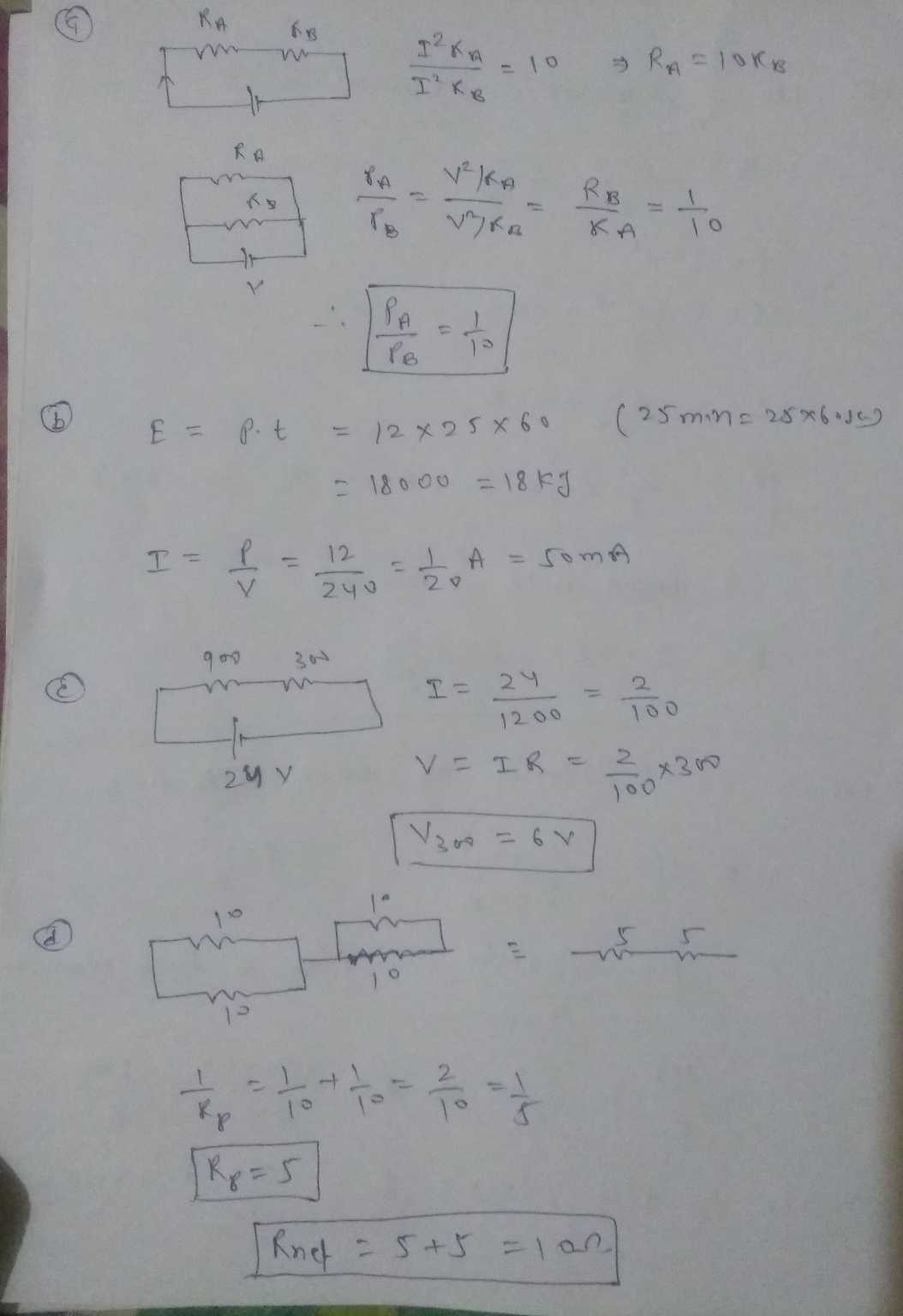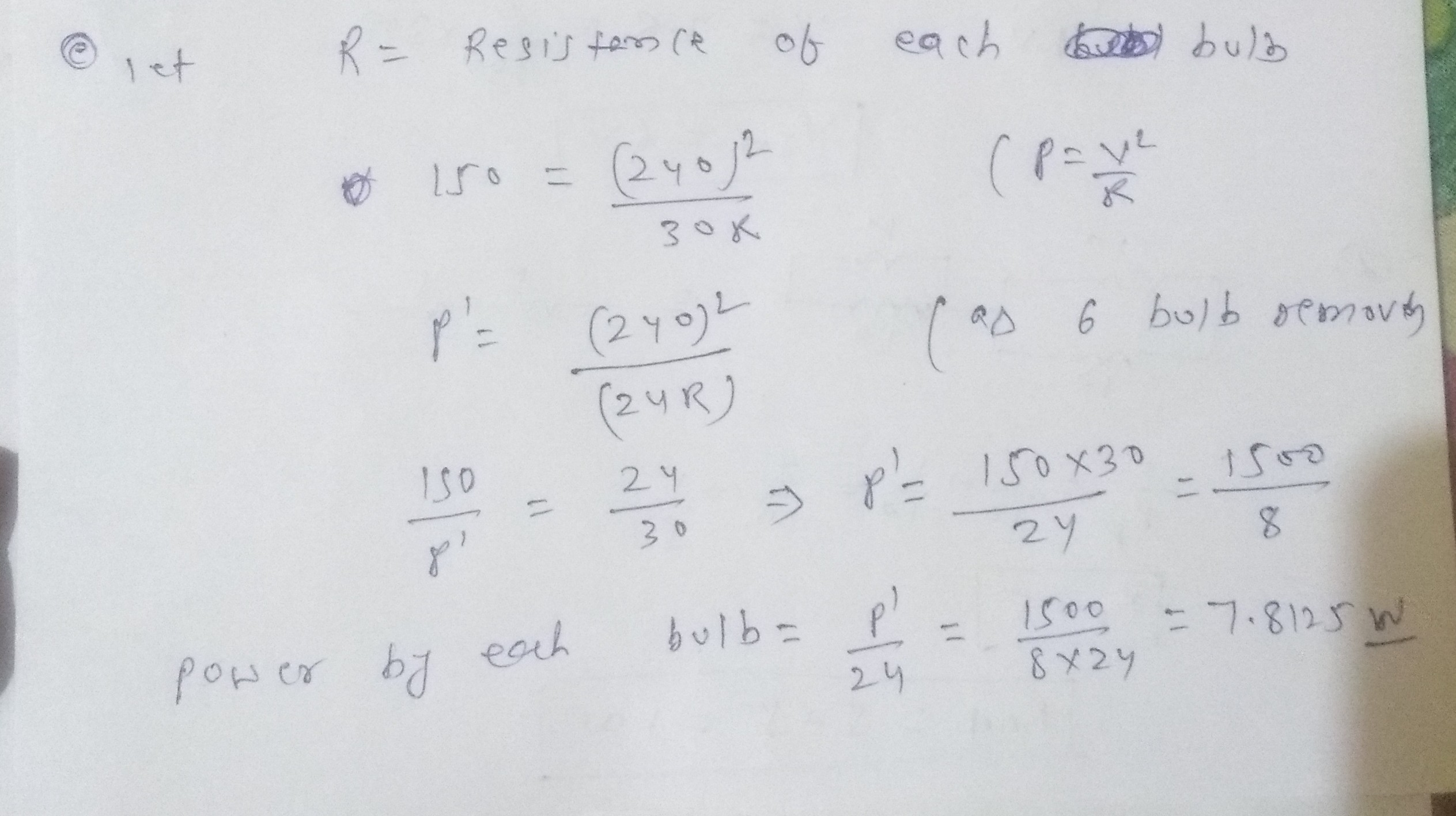#### Earn Coins

Coins can be redeemed for fabulous gifts.

Similar Homework Help Questions
• ### Two circuits A and B are connected in parallel to a 12 V battery which has...

Two circuits A and B are connected in parallel to a 12 V battery which has an internal resistance of 0.25 Ω. Circuit A consists of two resistors 1 Ω and 5 Ω connected in series. Circuit B consists of two resistors 4 Ω and 7 Ω connected in parallel. Calculate the currents flowing through and the voltages across each of the five resistors (the four resistors of circuits A and B and the internal resistance). Calculate the power dissipated...

• ### Two resistors are connected in parallel across an ideal 12.0 V battery. Resistor A has a...

Two resistors are connected in parallel across an ideal 12.0 V battery. Resistor A has a value of 28 Ω and resistor B carries a current of 0.2505 A. What is the total power dissipated in the circuit?Two resistors are connected in parallel across an ideal 12.0 V battery. Resistor A has a value of 28 Ω and resistor B carries a current of 0.2505 A. What is the total power dissipated in the circuit?

• ### (a) What is the equivalent resistance of six resistors connected in series with a 16.0-V battery...

(a) What is the equivalent resistance of six resistors connected in series with a 16.0-V battery if each resistor has a value of 23.0 Ω? (answer in Ω) (b) Determine the current flowing through each of the six resistors. (answer in A) (c) If the six resistors were instead connected in parallel across the battery, what would be the equivalent resistance? (answer in Ω) (d) Determine the current through each resistor for this parallel connection (answer in A)

• ### Three resistors are arranged as above. Ra=1.501, RB=3.0022 and Rc=5.5012. The battery has a potential of 18.0V. A. What...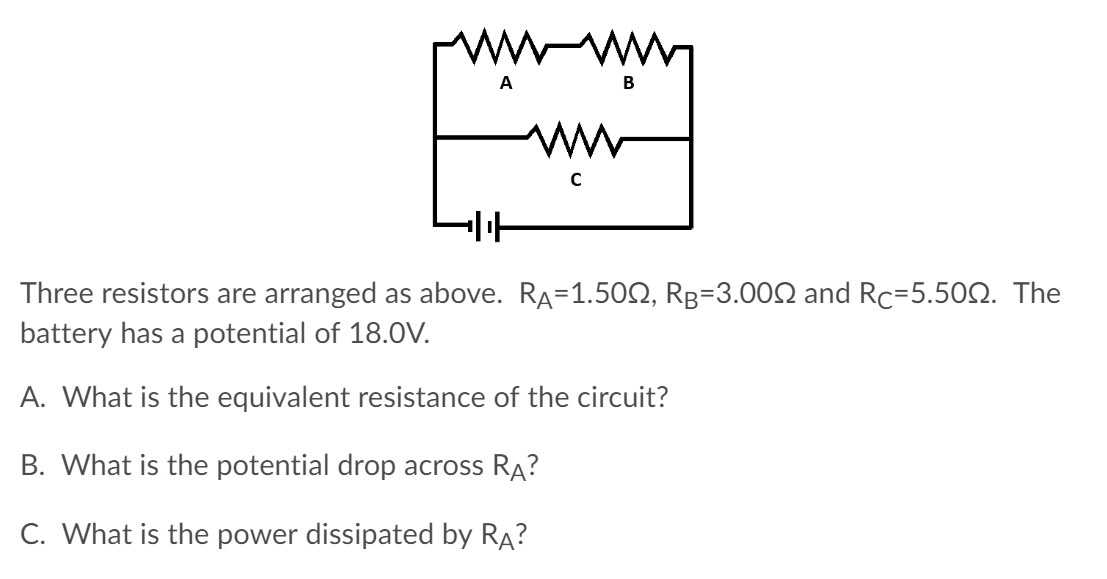Three resistors are arranged as above. Ra=1.501, RB=3.0022 and Rc=5.5012. The battery has a potential of 18.0V. A. What is the equivalent resistance of the circuit? B. What is the potential drop across RA? C. What is the power dissipated by RA?

• ### Resistors R1 = 4on and R2 = 60Ω are connected series and the combination is parallel...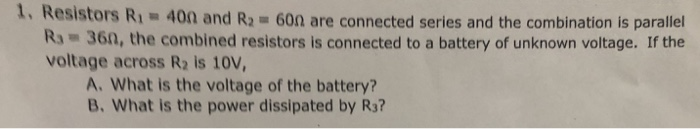Resistors R1 = 4on and R2 = 60Ω are connected series and the combination is parallel Ra 36n, the combined resistors is connected to a battery of unknown voltage. If the voltage across R2 is 10V, A. What is the voltage of the battery? B. what is the power dissipated by R

• ### Three 4.3 Ω resistors are connected in series with a 16.0 V battery. Find the following....

Three 4.3 Ω resistors are connected in series with a 16.0 V battery. Find the following. (a) the equivalent resistance of the circuit (b) the current in each resistor (c) Repeat for the case in which all three resistors are connected in parallel across the battery. equivalent resistance ______ current in each resistor _______

• ### Three 4.3 Ω resistors are connected in series with a 16.0 V battery. Find the following....

Three 4.3 Ω resistors are connected in series with a 16.0 V battery. Find the following. (a) the equivalent resistance of the circuit (b) the current in each resistor (c) Repeat for the case in which all three resistors are connected in parallel across the battery. equivalent resistance ______ current in each resistor _______

• ### Two resistors A and B are connected in series to a 6.0-V battery; the voltage across...

Two resistors A and B are connected in series to a 6.0-V battery; the voltage across resistor A is 2.0 V. When A and B are connected in parallel across a 6.0-V battery, the current through B is 2.0 A. What is the resistance of resistor A?

• ### Two resistors are connected in series with a battery whose EMF is 35 V as shown...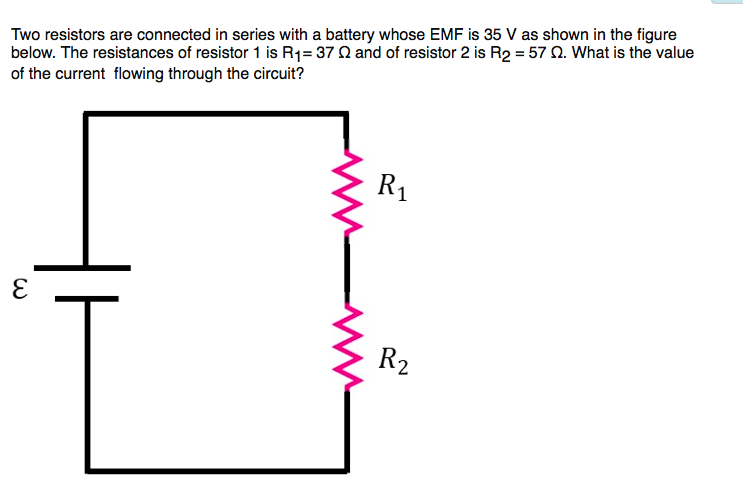Two resistors are connected in series with a battery whose EMF is 35 V as shown in the figure below. The resistances of resistor 1 is R1=37 and of resistor 2 is R2 = 57 2. What is the value of the current flowing through the circuit? R1 E R2 In the figure below bulb B is brighter than bulb C which is brighter than bulb A. Rank the magnitudes of the potential differences (voltages) across the bulbs, largest first...

• ### When unequal resistors are connected in series across an ideal battery, A) the same power is...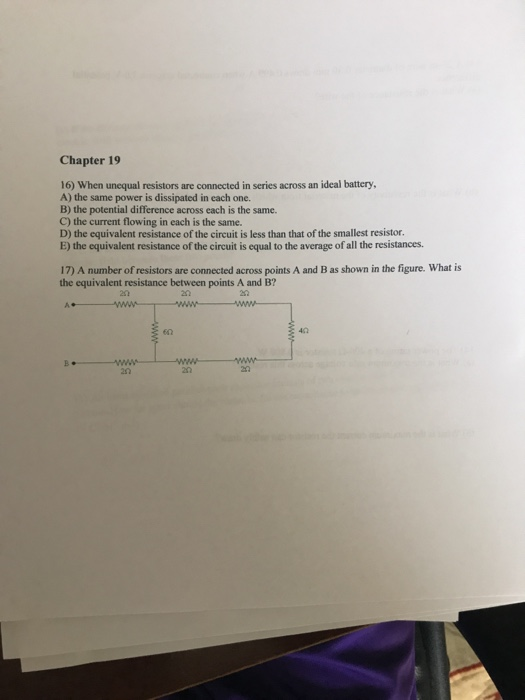When unequal resistors are connected in series across an ideal battery, A) the same power is dissipated in each one. B) the potential difference across each is the same. C) the current flowing in each is the same. D) the equivalent resistance of the circuit is less than that of the smallest resistor. E) the equivalent resistance of the circuit is equal to the average of all the resistances. A number of resistors are connected across points A and B...